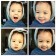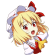# 计数排序算法初探

 a c {5,3,2,6,3,8,2} {0,0,0,0,1,0,0,0} {5,3,2,6,3,8,2} {0,0,1,0,0,0,0,0} {5,3,2,6,3,8,2} {0,1,1,0,1,0,0,0} {5,3,2,6,3,8,2} {0,1,1,0,1,1,0,0} {5,3,2,6,3,8,2} {0,1,2,0,1,1,0,0} {5,3,2,6,3,8,2} {0,1,2,0,1,1,0,1} {5,3,2,6,3,8,2} {0,2,2,0,1,1,0,1}

• 统计元素个数
• 累加计数来确定元素位置
• 每放一个数对应的计数建议
• 循环进行，直到排序结束

#include <stdio.h>

int main(int argc, char const *argv[])
{
const int NUM=10;	//Define the array constant equals 10

int input[NUM],output[NUM],temp;   //Define the necessary array
for (int i = 0; i < NUM; ++i)
{
scanf("%d",&input[i]);		//Initialize the input array (Use the user input)
}
for (int j = 0; j < 100; ++j)
{
temp[j]=0;					//Initialize the temp array (Use 0)
}
for (int k = 0; k < NUM; ++k)
{
temp[input[k]]=temp[input[k]]+1;	//Traverse the array and calculate the numbers of every elements
}
for (int l = 1; l < 100; ++l)
{
temp[l]=temp[l]+temp[l-1];			//Count the number which less than every elements
}
for (int m = NUM-1; m >= 0; m--)
{
output[temp[input[m]]]=input[m];	//Export the result to the OUTPUT array
temp[input[m]]=temp[input[m]]-1;
}
for (int n = 1; n <= NUM; ++n)
{
printf("%d\n",output[n] );		//Print all the array
}
return 0;
}

Wikipedia,计数排序

T.H.Cormen, C.E.Leiseron, R.L.Revest, C.Stein——算法导论,108-109.

## 《计数排序算法初探》上有2条评论

1.Captain说道：

那相比其他排序，它的优势是什么？

1.hcl说道：

速度应该会快一点（但这种差距在今天的计算机上已经很难体现了），相比于需要多出比较才能排序的算法，计数排序只要几遍遍遍历就能排完了。